# How Computers Math: AST

The answer to how a computer solves a math problem is not obvious, hence why I’m writing this and you’re reading it. I assume you know about order-of-operations and have used a complex calculator. First, let’s look at why math is not so straight-forward:

Solve this expression: $$8 + 2 / 2 - 3$$

Hopefully you got 6. You cannot solve this by purely going left-to-right. Do the division first, then read left-to-right. Computers must do the same thing except: how does the computer know what parts to do first? And the expressions can get more complicated: $$(1 + 3^2) / 2$$

Someone smarter than me came up with abstract syntax trees (AST). I’ll stop you now if you are thinking about the green and brown things that we use to make paper. A tree, in computer science, is a way of organizing pieces of information. It’s built using nodes, where each node can have any number of things attached to it but can only be attached to one thing. It looks like the branches of a tree. Its fall right now, so pause and look out your window at the naked trees.

Since my description is a little abstract, look at this picture.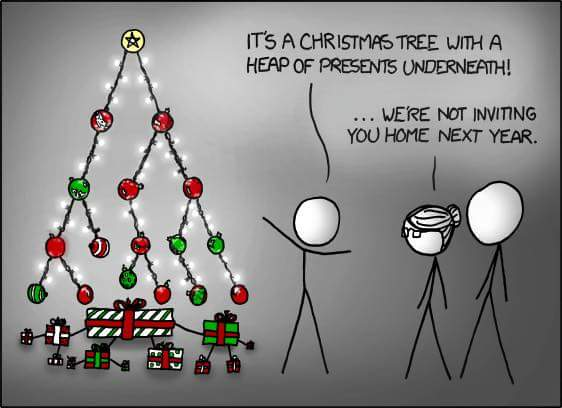The ornaments are nodes. The star is called the root. If a node has no children, it’s called a leaf. You might notice that a computer science tree looks more like an upside-down real-life tree. Just a few more terms to cover and then we can talk about solving the math problems. Here is a tree that I’ll use for some examples: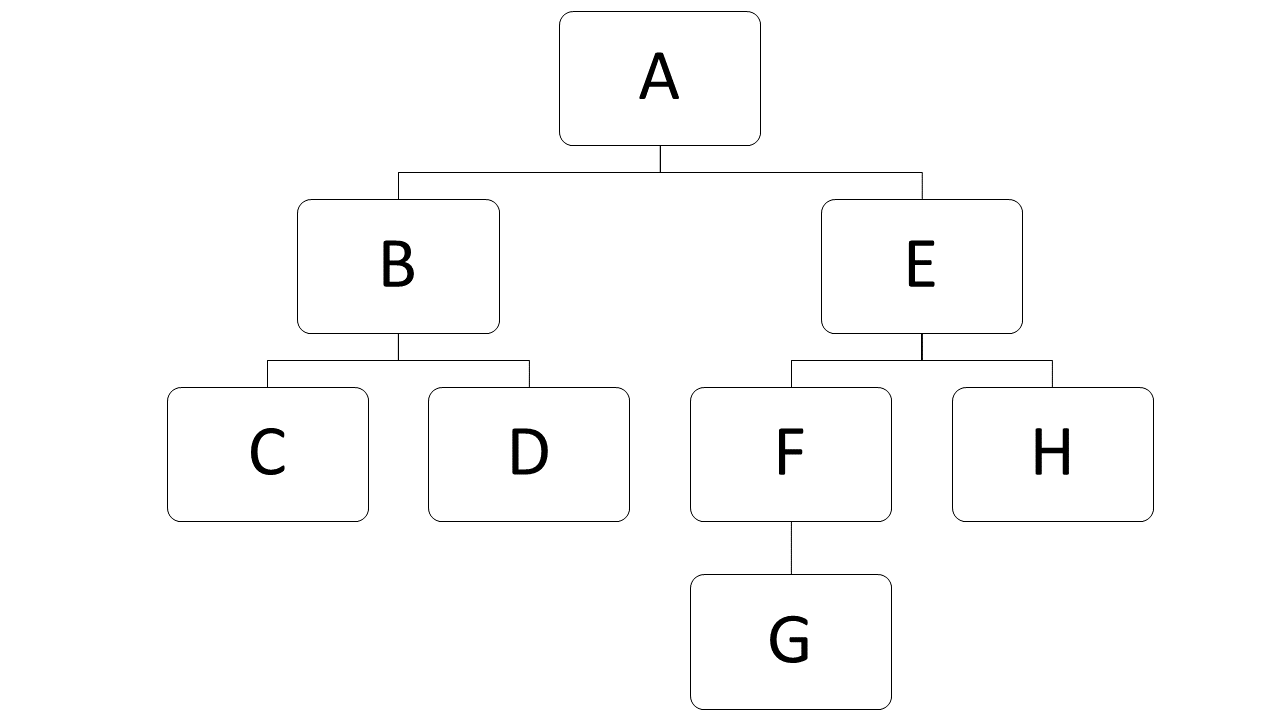Traversing a tree is when your code visits all the nodes. For example, to count the number of green ornaments, we must visit every node, check if it’s green, and if it is, make a tally mark. When traversing a tree, we start at the root node, but there are a few ways to go from there. We could look at the node and then look at its left child and then its right child. Following that path, here is the order in which we visit all the nodes for my example tree:

$$A, B, C, D, E, F, G, H$$

We could also traverse it by looking at the node’s left child, then the right, then the node itself. Here is the order in which we visit the nodes:

$$C, D, B, G, F, H, E, A$$

When you traverse a tree starting with a child, you end up starting at the bottom of the tree and working your way up.

## How a Human Solves the Tree

To solve a math problem, the computer builds a tree. The leaf nodes hold the numbers, and the parent nodes hold the operators. Here is an example for the expression 2 + 2 * 3: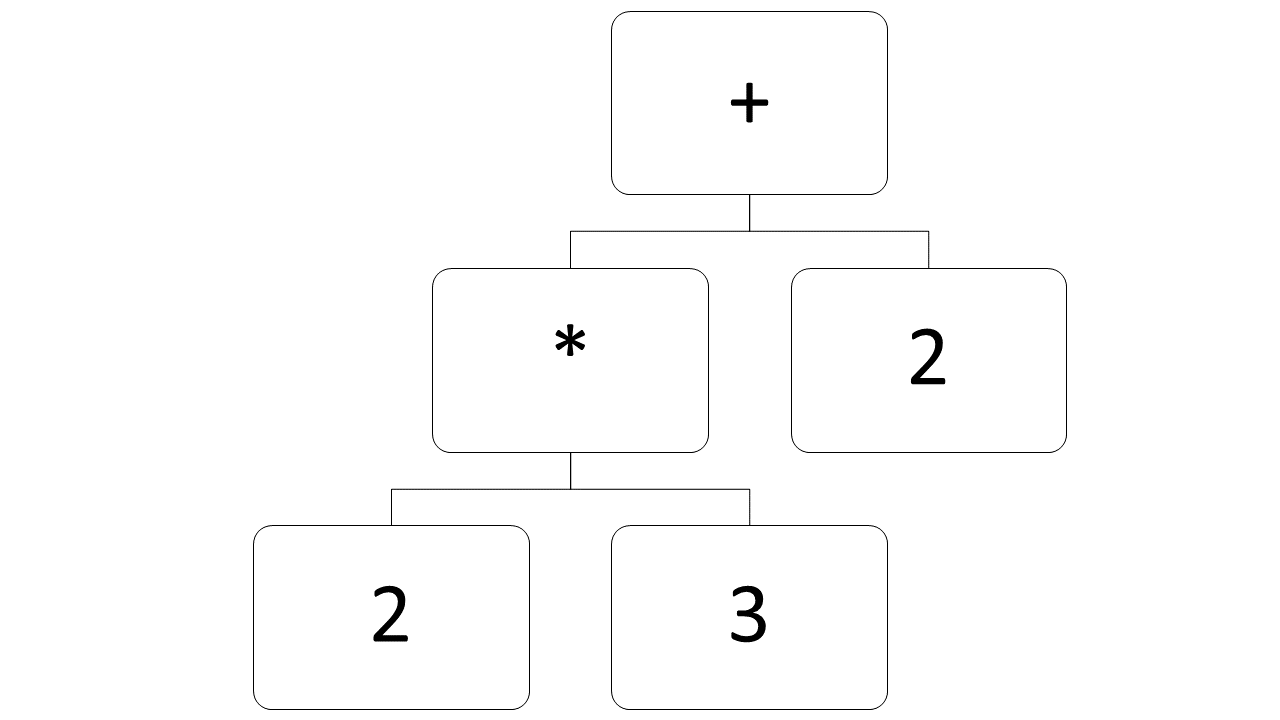Building the tree is outside the scope of this post. It involves writing a parser and defining rules on where to put the numbers and operators. Maybe I’ll do that for a future post? Now that you’ve got your tree, a human would solve it by reading the left child, the parent, and then the right, solving that simple expression, and putting the answer in the parent node. We’d start by looking at the bottom children and their parent.

Solve 2 * 3 and put it in the parent node.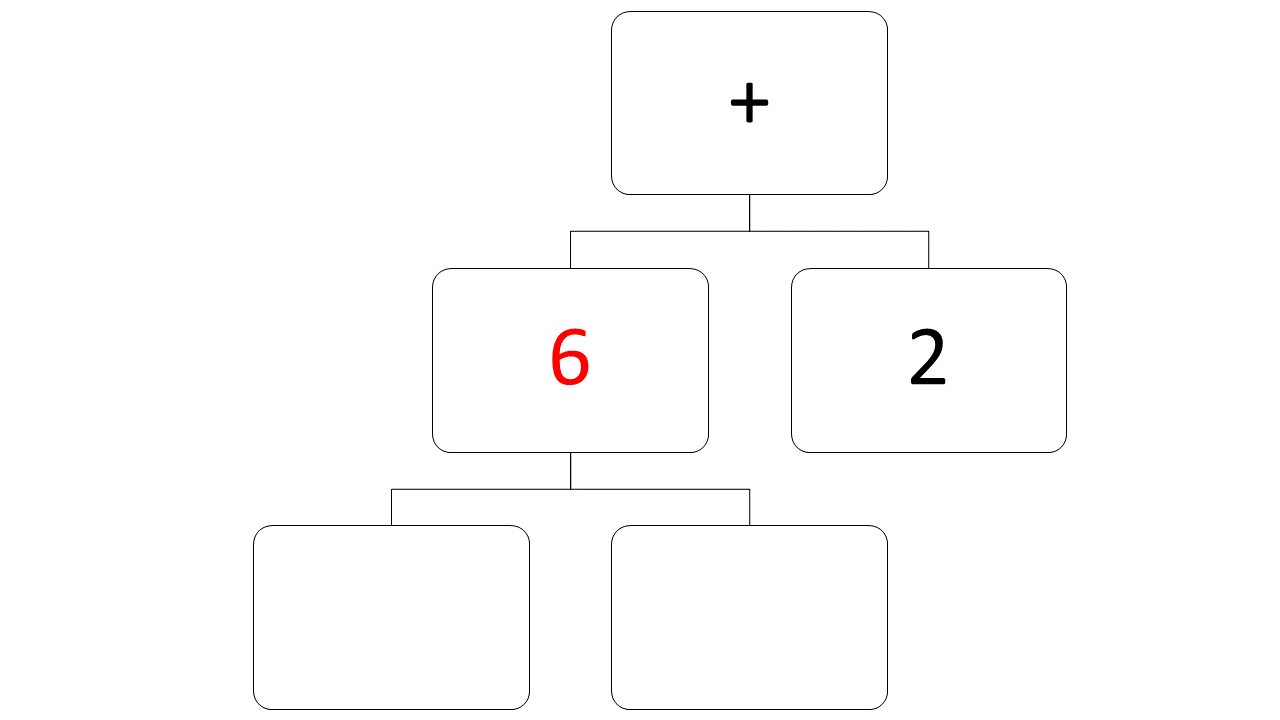Do the same thing for the next level up on the tree.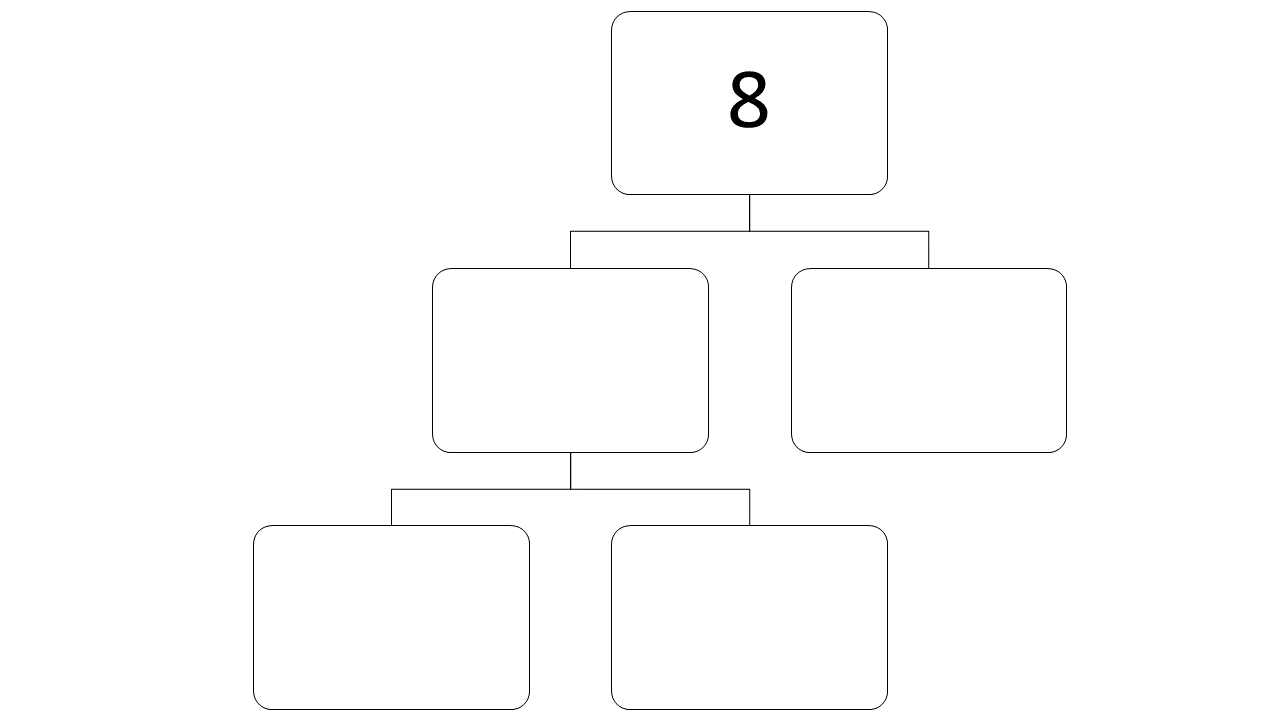## How a Computer Solves the Tree

When a computer solves a simple math expression, it doesn’t read it the same way a person does. People read 1 + 2 but computers read + 1 2. (That's just how x86 CPUs work) Here is the assembly code for solving 1 + 2 and putting the answer in memory:

ADC RAX, 1, 2

I use Intel assembly, so the destination register comes after the opcode.

Here's the part that gets me out of bed in the morning to write code. If you traverse the tree differently, you get the code to solve the tree. Assembly code requires you to read parent node, left node, then right node. There is a catch, unlike my earlier example, you must start at the bottom-most nodes. Purely following parent, left, right, gives you this invalid code:

ADC RAX, IMUL RBX, 2, 3, RBX, 2

For that AST we solved earlier, starting at the bottom-most parent and using RAX as the memory where we put the answer, here is the code:

IMUL RBX, 2, 3,
ADC RAX, RBX, 2

The answer is in RAX now.

For another example, let’s look at Adobe PostScript. Instead of discreet pieces of memory like RAX, RBX, etc., PostScript stacks the numbers on top of each other. When you do an operation, the computer pops the top two numbers off, does the operation, and puts the answer on top of the stack. (It’s called a stack machine) You need to traverse the tree so that you get code like this:

1 2 add

Traverse the tree going left node, right node, parent node. Here is the PostScript code you get for our example tree:

2 3 mul
2 add

The answer is on top of the stack.

If you’re looking for something more complicated to try, here is an expression and the AST for it. Solve it or write the code for it.

$$1 + \sqrt{9} + \frac{14}{2}$$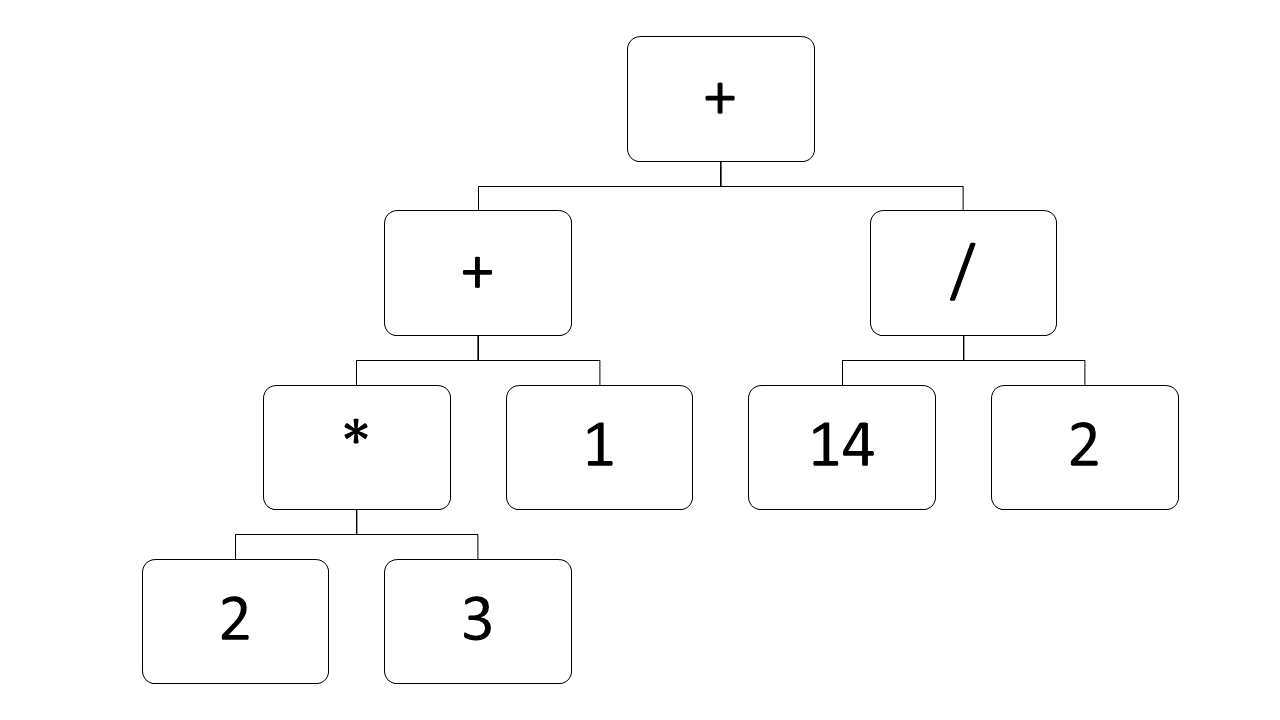If you’re writing assembly, you must start at the bottom. It’s like solving each level of the tree simultaneously.

IMUL RCX, 2, 3
ADC RAX, RBX, RDX